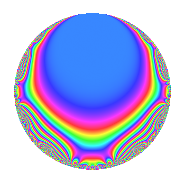# Properties

 Label 630.2.d.bLevel 630 Weight 2 Character orbit 630.d Analytic conductor 5.031 Analytic rank 0 Dimension 4 CM No Inner twists 4

# Related objects

## Newspace parameters

 Level: $$N$$ = $$630 = 2 \cdot 3^{2} \cdot 5 \cdot 7$$ Weight: $$k$$ = $$2$$ Character orbit: $$[\chi]$$ = 630.d (of order $$2$$ and degree $$1$$)

## Newform invariants

 Self dual: No Analytic conductor: $$5.03057532734$$ Analytic rank: $$0$$ Dimension: $$4$$ Coefficient field: $$\Q(\sqrt{-2}, \sqrt{5})$$ Coefficient ring: $$\Z[a_1, \ldots, a_{7}]$$ Coefficient ring index: $$2^{2}$$ Sato-Tate group: $\mathrm{SU}(2)[C_{2}]$

## $q$-expansion

Coefficients of the $$q$$-expansion are expressed in terms of a basis $$1,\beta_1,\beta_2,\beta_3$$ for the coefficient ring described below. We also show the integral $$q$$-expansion of the trace form.

 $$f(q)$$ $$=$$ $$q - q^{2} + q^{4} + \beta_{3} q^{5} + ( -\beta_{1} - \beta_{3} ) q^{7} - q^{8} +O(q^{10})$$ $$q - q^{2} + q^{4} + \beta_{3} q^{5} + ( -\beta_{1} - \beta_{3} ) q^{7} - q^{8} -\beta_{3} q^{10} + 4 \beta_{1} q^{11} + 2 \beta_{3} q^{13} + ( \beta_{1} + \beta_{3} ) q^{14} + q^{16} + \beta_{2} q^{17} -\beta_{2} q^{19} + \beta_{3} q^{20} -4 \beta_{1} q^{22} + 4 q^{23} + 5 q^{25} -2 \beta_{3} q^{26} + ( -\beta_{1} - \beta_{3} ) q^{28} + 2 \beta_{1} q^{29} -2 \beta_{2} q^{31} - q^{32} -\beta_{2} q^{34} + ( -5 - \beta_{2} ) q^{35} + 7 \beta_{1} q^{37} + \beta_{2} q^{38} -\beta_{3} q^{40} + 2 \beta_{3} q^{41} + \beta_{1} q^{43} + 4 \beta_{1} q^{44} -4 q^{46} -3 \beta_{2} q^{47} + ( 3 + 2 \beta_{2} ) q^{49} -5 q^{50} + 2 \beta_{3} q^{52} + 4 q^{53} + 4 \beta_{2} q^{55} + ( \beta_{1} + \beta_{3} ) q^{56} -2 \beta_{1} q^{58} + 2 \beta_{3} q^{59} -3 \beta_{2} q^{61} + 2 \beta_{2} q^{62} + q^{64} + 10 q^{65} + 5 \beta_{1} q^{67} + \beta_{2} q^{68} + ( 5 + \beta_{2} ) q^{70} + \beta_{1} q^{71} + 6 \beta_{3} q^{73} -7 \beta_{1} q^{74} -\beta_{2} q^{76} + ( 8 - 4 \beta_{2} ) q^{77} + 6 q^{79} + \beta_{3} q^{80} -2 \beta_{3} q^{82} + 4 \beta_{2} q^{83} + 5 \beta_{1} q^{85} -\beta_{1} q^{86} -4 \beta_{1} q^{88} -2 \beta_{3} q^{89} + ( -10 - 2 \beta_{2} ) q^{91} + 4 q^{92} + 3 \beta_{2} q^{94} -5 \beta_{1} q^{95} + ( -3 - 2 \beta_{2} ) q^{98} +O(q^{100})$$ $$\operatorname{Tr}(f)(q)$$ $$=$$ $$4q - 4q^{2} + 4q^{4} - 4q^{8} + O(q^{10})$$ $$4q - 4q^{2} + 4q^{4} - 4q^{8} + 4q^{16} + 16q^{23} + 20q^{25} - 4q^{32} - 20q^{35} - 16q^{46} + 12q^{49} - 20q^{50} + 16q^{53} + 4q^{64} + 40q^{65} + 20q^{70} + 32q^{77} + 24q^{79} - 40q^{91} + 16q^{92} - 12q^{98} + O(q^{100})$$

Basis of coefficient ring in terms of a root $$\nu$$ of $$x^{4} + 6 x^{2} + 4$$:

 $$\beta_{0}$$ $$=$$ $$1$$ $$\beta_{1}$$ $$=$$ $$($$$$\nu^{3} + 4 \nu$$$$)/2$$ $$\beta_{2}$$ $$=$$ $$($$$$\nu^{3} + 8 \nu$$$$)/2$$ $$\beta_{3}$$ $$=$$ $$\nu^{2} + 3$$
 $$1$$ $$=$$ $$\beta_0$$ $$\nu$$ $$=$$ $$($$$$\beta_{2} - \beta_{1}$$$$)/2$$ $$\nu^{2}$$ $$=$$ $$\beta_{3} - 3$$ $$\nu^{3}$$ $$=$$ $$-2 \beta_{2} + 4 \beta_{1}$$

## Character Values

We give the values of $$\chi$$ on generators for $$\left(\mathbb{Z}/630\mathbb{Z}\right)^\times$$.

 $$n$$ $$127$$ $$281$$ $$451$$ $$\chi(n)$$ $$-1$$ $$-1$$ $$-1$$

## Embeddings

For each embedding $$\iota_m$$ of the coefficient field, the values $$\iota_m(a_n)$$ are shown below.

For more information on an embedded modular form you can click on its label.

Label $$\iota_m(\nu)$$ $$a_{2}$$ $$a_{3}$$ $$a_{4}$$ $$a_{5}$$ $$a_{6}$$ $$a_{7}$$ $$a_{8}$$ $$a_{9}$$ $$a_{10}$$
629.1
 − 2.28825i 2.28825i 0.874032i − 0.874032i
−1.00000 0 1.00000 −2.23607 0 2.23607 1.41421i −1.00000 0 2.23607
629.2 −1.00000 0 1.00000 −2.23607 0 2.23607 + 1.41421i −1.00000 0 2.23607
629.3 −1.00000 0 1.00000 2.23607 0 −2.23607 1.41421i −1.00000 0 −2.23607
629.4 −1.00000 0 1.00000 2.23607 0 −2.23607 + 1.41421i −1.00000 0 −2.23607
 $$n$$: e.g. 2-40 or 990-1000 Significant digits: Format: Complex embeddings Normalized embeddings Satake parameters Satake angles

## Inner twists

Char. orbit Parity Mult. Self Twist Proved
1.a Even 1 trivial yes
7.b Odd 1 yes
15.d Odd 1 yes
105.g Even 1 yes

## Hecke kernels

This newform can be constructed as the intersection of the kernels of the following linear operators acting on $$S_{2}^{\mathrm{new}}(630, [\chi])$$:

 $$T_{11}^{2} + 32$$ $$T_{23} - 4$$